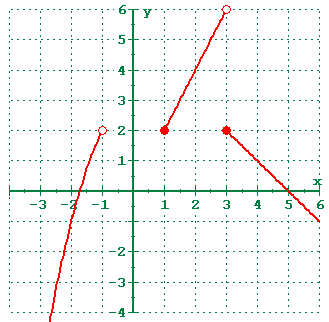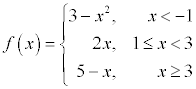# 1.3 - Functions

## Definitions

Relation
A rule that associates a value in the domain with a value in the range.
Function
A function is a relation (rule) that assigns each element in the domain to exactly one element in the range.
Domain
The set of all the values which may be input into a function. That is, the set of all the values the independent variable may assume. Graphically, the domain is the set of all the x-coordinates.
Range
The set of all the values which are output when the function is evaluated at all the input values from the domain. That is, the set of all the values the dependent variable may assume. Graphically, the range is the set of all the y-coordinates.
Independent Variable
Typically, the independent variable is x. However, the independent variable is the variable which is free to assume different values independently of the other variable. Most of what we're going to do in this class will only involve one independent variable, but realize that it is possible to have more than one independent variable.
Dependent Variable
Typically, the dependent variable is y. However, the dependent variable is the variable which is determined based on the value of the independent variable(s). If a function is written as y=3x+2, then y depends on x, but x doesn't depend on y (in the form it's written in). So, x is independent of y, but y is dependent of x.
Implied Domain
The set of all real numbers for which the expression is defined.

## How is a function different from a relation?

Here are some guidelines for determining whether a relation is a function or not.

1. Each and every element in the domain (x) must be matched with an element in the range (y).
2. Every element in the domain (x) can only be matched with one element in the range (y).
3. Different elements in the domain can go to the same element in the range. A y-coordinate may be repeated, but an x-coordinate may not.
4. Some values in the range don't have to be used at all

## Function Notation

Function notation is used to name functions for easy reference. Imagine if every function in the world had to start off with y=. Pretty soon, you would become confused about which y= you were talking about. This is the way antecedents work in English. If you just say "it" is red, you really don't have any clue which "it" you're talking about. You need some other way of naming things. Enter function notation.

### Function Definition

• f(x) = 3x + 2
• g(x,y) = x2 + 3y

In this example, the f is a function of x. That is, x is the independent variable, and the value of f depends on what x is. Also, g is a function of both x and y.

The notation f(x) does not mean f times x. It means the "value of f evaluated at x" or "value of f at x" or simply "f of x"

### Function Evaluation

f(3) = 3(3) + 2 = 9 + 2 = 11

f(3) does not mean f times 3. It means the "value of f evaluated when x is 3".

f(t) = 3(t) + 2 = 3t + 2

Whatever is in parentheses on the left side of the function (t in this case) is substituted for the value of the independent variable on the right side.

f(x+h) = 3(x+h) + 2 = 3x + 3h + 2

Every occurrence of the independent variable is replaced by the quantity in parentheses. A common mistake is to take a quantity and apply linear or affine transformations to it.

### f(x+h)

• f(x+h) does not equal f(x) + h = 3x+2 + h
• f(x+h) does not equal f(x) + f(h) = 3x+2 + 3h+2 = 3x + 3h + 4
• f(x+h) does equal 3(x+h) + 2 = 3x + 3h + 2

### f(3x)

• f(3x) does not equal 3 * f(x) = 3( 3x+2) = 9x + 6
• It does equal 3(3x) + 2 = 9x + 2

You also specify which function you want to use when you use function notation. Consider

g(2,1) = 22 + 3(1) = 4 + 3 = 7

Since the order of the independent variables in the original definition was x and then y, the function g is evaluated when x=2 and y=1.

g(1,2) is something completely different. In this case, x=1 and y=2.

g(1,2) = 12 + 3(2) = 1 + 6 = 7

Okay, so it's not completely different, but it is found in a different manner.

## Piecewise Definitions

Sometimes, functions are a little more complex than the simple functions we've described so far. If different rules are used for different values of the independent variable, then we can use a piecewise definition.Consider the function shown and the following evaluations.

f(2.1) = 2(2.1) = 4.2

Since 2.1 is in the interval [1,3), we use the second piece of the definition, f(x) = 2x.

f(-2) = 3 - (-2)2 =3 - 4 = -1

Since -2 is in the interval (-∞,-1), we use the first piece of the definition, f(x) = 3-x2

f(3) = 5 - (3) = 5 - 3 = 2

Since 3 is not included in the second piece, but it is included in the third piece on the interval [3,+∞) we use the third piece of the definition, f(x) = 5 - x.

f(0) is undefined

Since 0 doesn't fall into any of the domains, the function is undefined there.

When all of the domains are combined, the domain of the function f, is the set of all real numbers except [-1,1). You could also write it as (-∞,-1)U[1,+∞). The symbol U stands for the union of two sets.

## Piecewise Functions and the Calculator

You can put piecewise functions into the graphing calculator. The TI82 and TI83 calculators have a [Test] key obtained by pressing [2nd] [Math]. Under this key, you will find the different test operators (equal to, not equal to, greater than, greater than or equal to, less than, and less than or equal to).

When the calculator evaluates a test expression (x<1), it will return the value of 1 if the statement is true and the value of 0 if the statement is false. This works really well with multiplication, because multiplying by 1 won't change the expression, and multiplying by 0 will make the expression 0.

y = (1 - x2)*(x<-1)

Consider just the first part of the piecewise definition from above. If x<-1, then the calculator will return (1-x^2). If x is not less than negative one, then the calculator will return 0. That's not the proper value to return (it should be undefined), but it will graph on the x-axis and won't show up because the axis is already there. So, it has the semblance on graphing correctly.

The complete piecewise function can be defined for the calculator as:

y = (1-x2)*(x<-1) + 5x*(1≤x and x<3) + (5-3x)*(x≥3)

The "and" keyword can be found under [Test] Logic menu. Similarly, the ≤ and ≥ symbols can also be found under the [Test] menu. If you try to put in 1≤x<3 like we right, the calculator will interpret that as 1≤3 and always return true.

Be sure to use some kind of decimal setting or dot mode when you're viewing a piecewise function. Otherwise, you may get some weird results.

## Finding the Domain

### Implied Domain

We defined implied domain earlier. It is the set of all real numbers where the expression is defined.

1. Start off with all real numbers
2. Exclude any values of the independent variable which cause division by zero
3. Exclude any values of the independent variable which result in taking the square root of a negative number. Square root can be replaced with any even (4th, 6th, etc) root.

You do not need to state restrictions which result from the implied domain. In other words, if there is an (x-2) in the denominator, you do not need to state that x cannot be 2.

### Other Exclusions

Sometimes, you will need to exclude other values.

1. Problem Constraints - these are used in applications. Here are some examples
• If x is the length of the side of a triangle, then x can't be negative
• If x is the position on a 12' teeter totter at which the fulcrum must be positioned, then x must be between 0 and 12.
• If x is the number of people in a room, then x can't be negative.
2. Stated Restrictions - sometimes the problem will just come out and say you can't use a specific value, or that you can only use specific values. These aren't in the implied domain, so they need to be stated.

If you ever simplify a function, and a value which was in the implied domain is no longer in the implied domain, then it needs to become a stated restriction. Example, divide (x2-4) by (x-2). The implied domain is x cannot equal 2 because that would cause division by zero. However, if you factor the numerator as (x-2)(x+2), then (x-2) in the numerator divides out with the (x-2) in the denominator and you're left with just (x+2). The fact that x cannot be 2 is no longer implied by a simple x+2 in the numerator, so you must now state that x cannot be 2.

## Combining Domains

When you have a function which is a composition of several pieces, the values in the domain must be able to be used in all parts of the function. Let's say the numerator has a square root of x in it (so that x must be non-negative) and the denominator has an x-2 in it (so that x cannot be 2). When you combine those domains, you get all values of x that are non-negative except 2.

In other words, the domain for the function is the intersection of all the domains of the individual parts.

Now, let's say the numerator has a square root of x in it (so that x must be non-negative) and the denominator has an x+2 in it (so that x cannot be -2). When you combine those domains, you get all values of x that are non-negative except -2. Well, you don't need to state that x cannot be -2 since you've already said that x is non-negative. So, in this case, the domain would be all non-negative values of x.